Examples

Chapter 8 Class 10 Introduction to Trignometry
Serial order wise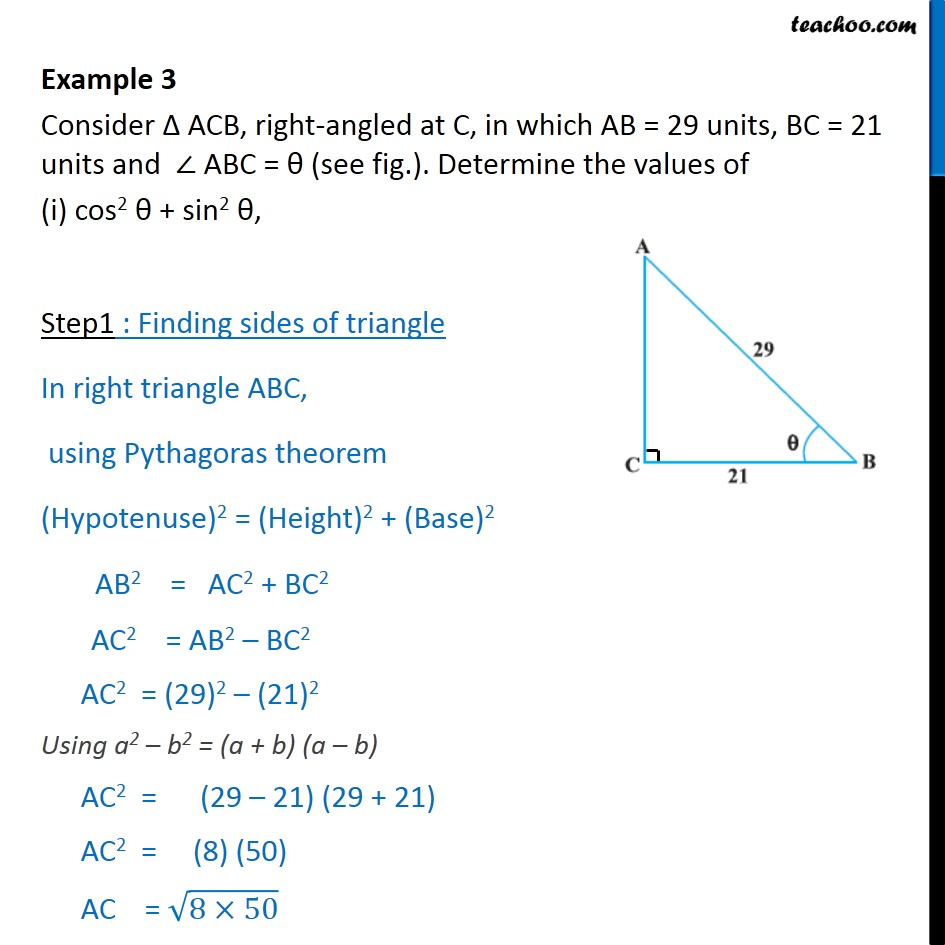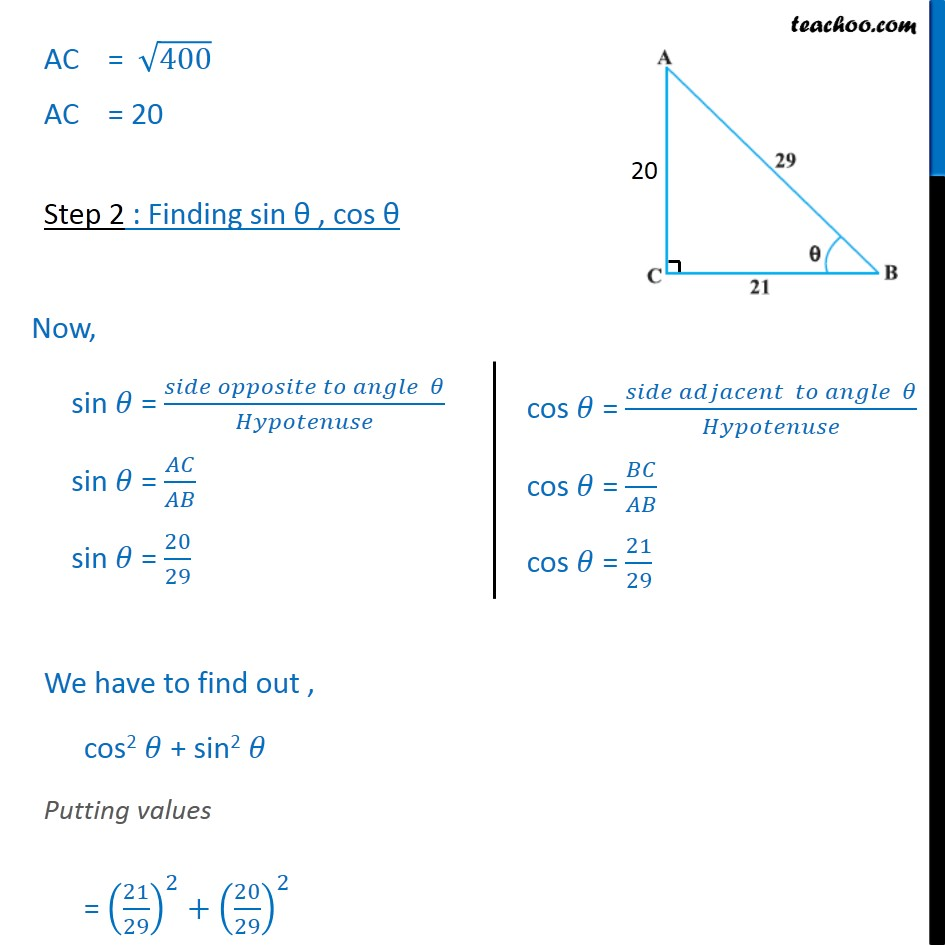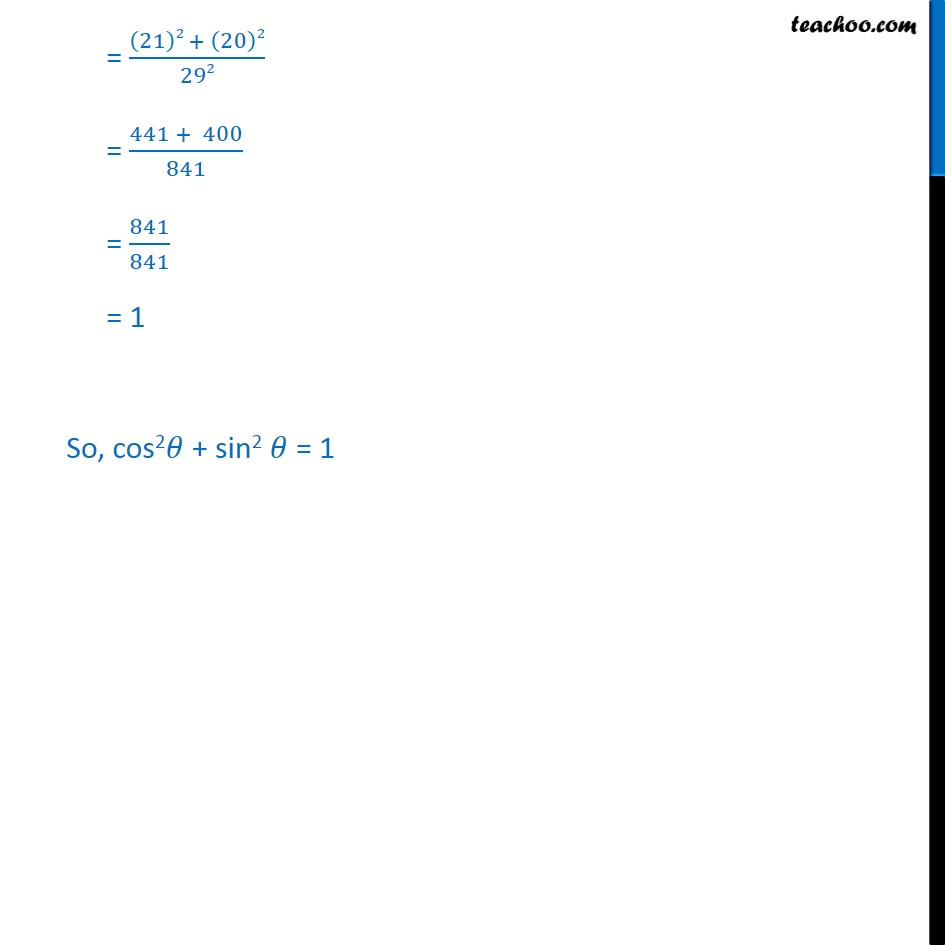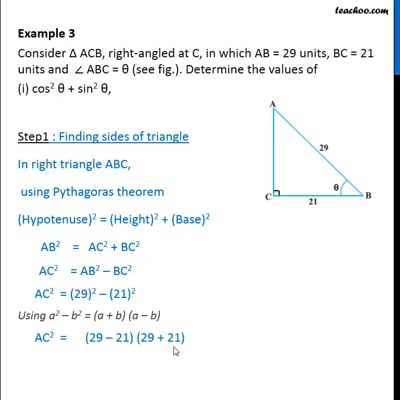This video is only available for Teachoo black users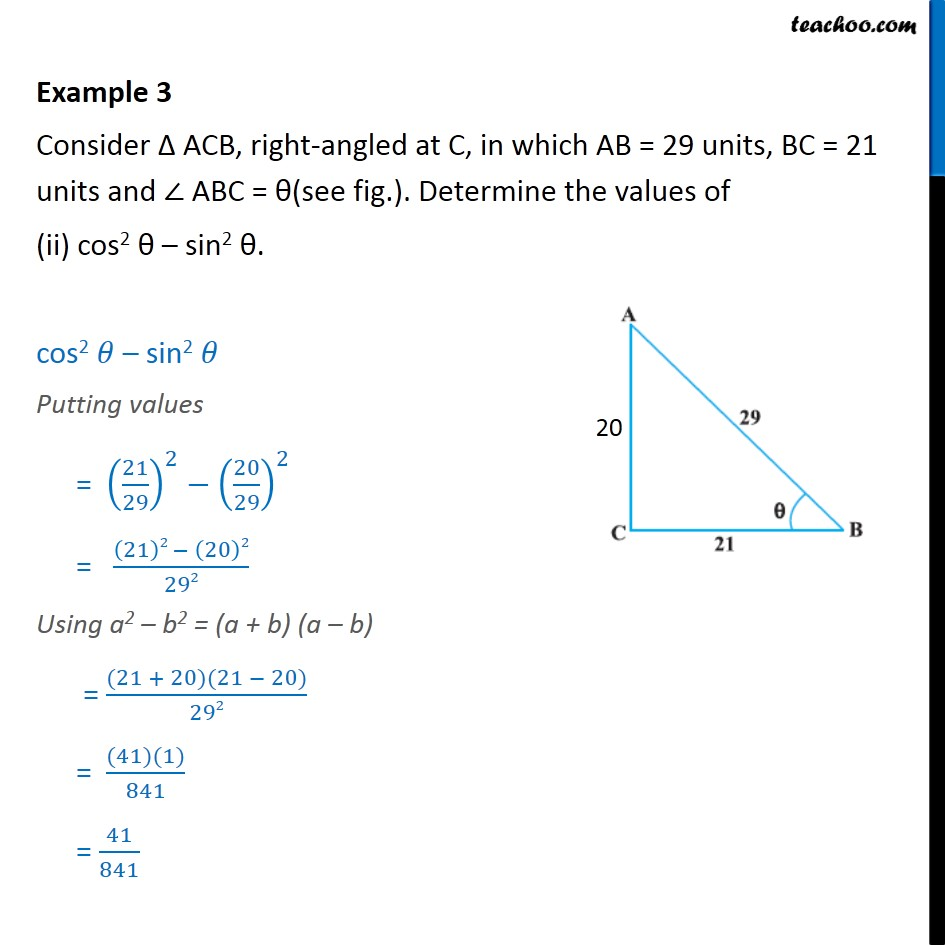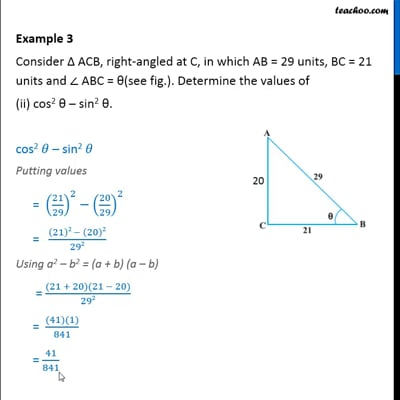This video is only available for Teachoo black users

Solve all your doubts with Teachoo Black (new monthly pack available now!)

### Transcript

Example 3 Consider ACB, right-angled at C, in which AB = 29 units, BC = 21 units and ABC = (see fig.). Determine the values of (i) cos2 + sin2 , Step1 : Finding sides of triangle In right triangle ABC, using Pythagoras theorem (Hypotenuse)2 = (Height)2 + (Base)2 AB2 = AC2 + BC2 AC2 = AB2 BC2 AC2 = (29)2 (21)2 Using a2 b2 = (a + b) (a b) AC2 = (29 21) (29 + 21) AC2 = (8) (50) AC = (8 50) AC = 400 AC = 20 Step 2 : Finding sin , cos We have to find out , cos2 + sin2 Putting values = (21/29)^2+(20/29)^2 = ((21)2 + (20)2)/292 = (441 + 400)/841 = 841/841 = 1 So, cos2 + sin2 = 1 Example 3 Consider ACB, right-angled at C, in which AB = 29 units, BC = 21 units and ABC = (see fig.). Determine the values of (ii) cos2 sin2 . cos2 sin2 Putting values = (21/29)^2 (20/29)^2 = ((21)2 (20)2)/292 Using a2 b2 = (a + b) (a b) = ((21 + 20)(21 20))/292 = ((41)(1))/841 = 41/841